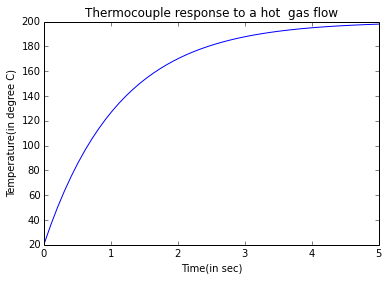# Chapter 1 - "Introduction"¶

### Example 1.1, Page number: 13¶

In :
from __future__ import division
import math

#Variables
k=35;                                      #Thermal  Conductivity,  [W/m*K]
T1=110                                     #  Temperature  of  front[C]
T2=50;                                     #  Temperature  of  back,[C]
A=0.4                                      #area  of  slab,[m**2]
x=0.03;                                    #Thickness  of  slab,[m]

#Calculations
q=-k*(T2-T1)/(1000*x);                     #formula  for  heat  flux[KW/m^2]
Q=q*A;                                     #formula  for  heat  transfer  rate[KW]

#Results
print "Heat flux is:",q,"KW/m^2\n"
print "Heat transfer rate is:",Q,"KW \n"

Heat flux is: 70.0 KW/m^2

Heat transfer rate is: 28.0 KW



### Example 1.2, Page number: 16¶

In :
from __future__ import division
import math
from sympy import solve,symbols

#Variables
x=symbols('x');
k1=372;                                 #  Thermal  Conductivity  of  slab,W/m*K
x1=0.003;                               #  Thickness  of  slab,m
x2=0.002                                #  Thickness  of  steel,m
k2=17;                                  #  Thermal  Conductivity  of  steel,W/m*K
T1=400;                                 #  Temperature  on  one  side,C
T2=100                                  #Temperature  on  other  side,C

#Calculations
Tcu=solve(x+2*x*(k1/x1)*(x2/k2)-(T1-T2),x);
#q=k1*(Tcu/x1)=k2*(Tss/x2);
Tss  =  Tcu*(k1/x1)*(x2/k2);         #  formula for temperature gradient in steel side
Tcul=T1-Tss;
Tcur=T2+Tss;
q=k2*Tss/(1000*x2);                     #  formula for heat conducted, kW\m^2

#Results
print "Temperature on left copper side is :",round(Tcul,3),"C\n"
print "Temperature on right copper  side is :",round(Tcur,3),"C\n"
print "Heat conducted through the wall is :",round(q,3),"kW\m^2\n"
print "Our initial approximation was accurate within a few percent."

Temperature on left copper side is : 254.971 C

Temperature on right copper  side is : 245.029 C

Heat conducted through the wall is : 1232.749 kW\m^2

Our initial approximation was accurate within a few percent.


### Example 1.3, Page number: 22¶

In :
from __future__ import division
import math

#Variables
q1=6000;                                          #Heat  flux,  W*m**-2
T1=120;                                           #Heater  Temperature,  C
T2=70;                                            #final  Temperature  of  Heater, C
q2=2000;                                          #final  heat  flux, W*m**-2

#Calculations
h=q1/(T1-T2)                                      #formula  for  average  heat  transfer  cofficient
Tnew=T2+q2/h;                                     #formula  for  new  Heater  temperature, C

#Results
print "Average Heat transfer coefficient is:",h,"W/(m^2*K)\n"
print "New Heater Temperature is:",round(Tnew,3),"C\n"

Average Heat transfer coefficient is: 120.0 W/(m^2*K)

New Heater Temperature is: 86.667 C



### Example 1.4, Page number: 25¶

In :
from __future__ import division
import math
from numpy import array
from numpy import linspace
import matplotlib.pyplot as plt
from pylab import *
%matplotlib inline

#Variables
h=250;                                  #Heat Transfer  Coefficient,  W/(m**2*K)
k=45;                                   #Thermal  Conductivity,  W/(m*K)
c=180;                                  #Heat  Capacity,  J/(kg*K)
a=9300;                                 #density,  kg/m**3
T1=200;                                 #temperature,  C
t1=linspace(0,5,50);                    #defining time interval of 0.1 seconds
T=linspace(0,5,50);
i=0;

#Calculations
while i<50:
T[i]=T1-c*math.exp(-t1[i]/((a*c*D)/(6*h)));      #Calculating temperature at each time in degree C
i=i+1;

plt.plot(t1,T);
plt.xlabel("Time(in sec)");
plt.ylabel("Temperature(in degree C)");
plt.title("Thermocouple response to a hot  gas flow");
plt.show();

Bi  =  h*(D/2)/k;                       #biot  no.

#Results
print "The value of Biot no for this thermocouple is",round(Bi,5);
print "Bi is <0.1 and hence the thermocouple could be considered as a lumped heat capacity system and the assumption taken is valid.\n"The value of Biot no for this thermocouple is 0.00278
Bi is <0.1 and hence the thermocouple could be considered as a lumped heat capacity system and the assumption taken is valid.



### Example 1.5, Page number: 32¶

In :
from __future__ import division
import math
from sympy import solve,symbols

#Variables
x=symbols('x');
T1=293;                                    #Temperature  of  air  around  thermocouple,  K
T2=373;                                    #Wall  temperature,  K
h=75;                                      #Average  Heat  Transfer  Coefficient,  W/(m**2*K)
s=5.67*10**-8;                             #stefan  Boltzman  constant,  W/(m**2*K**4)

#Calculations
x=solve((h*(x-T1)+s*(x**4-T2**4)),x);	   #Calculating Thermocouple Temperature, K
y=x-273;				                   #Thermocouple Temperature, C

#Results
print "Thermocouple Temperature is :",round(y,3),"C\n"

Thermocouple Temperature is : 28.395 C



### Example 1.6, Page number: 34¶

In :
from __future__ import division
import math
from sympy import solve,symbols

#Variables
x=symbols('x');
e=0.4;                                             #emissivity
T1=293;                                            #Temperature  of  air  around  Thermocouple,  K
T2=273;                                            #wall  Temperature,  K
h=75;                                              #Average  Heat  Transfer  Coefficient,  W/(m**2*K)
s=5.6704*10**-8;                                   #stefan  Boltzman  constant,  W/(m**2*K**4)

#Calculations
z=solve(((s*e*((373)**4 - (x)**4)) - h*(x-293)),x);	#Calculating Thermocouple Temperature, K
y=z-273;					                        #Thermocouple Temperature, C

'''NOTE: Equation written is absolutely correct and solving this equation
should give real result as: 296.112 i.e. 23.112 C, but somehow python is giving wrong result.'''

#Results
print "Thermocouple Temperature is :",round(y,1),"C \n"

Thermocouple Temperature is : 25.9 C